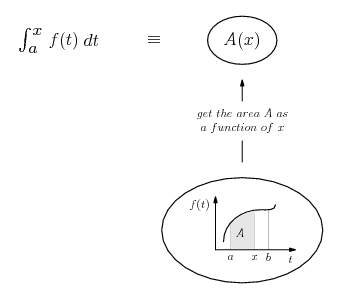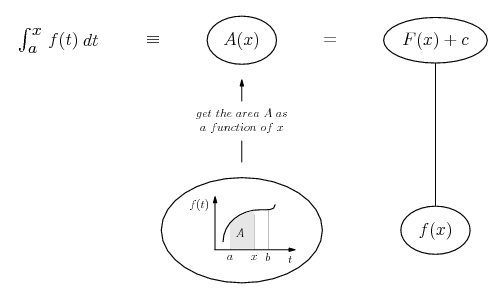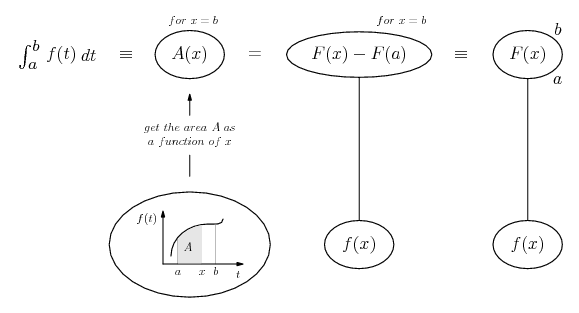# Thread: Definite Integrals..how are they different from...

1. ## Definite Integrals..how are they different from...

I'm having trouble understanding the difference between "finding area of a region under a curve by limit definition" and by "definite integrals."

To me, the definite integral process is basically the same...I honestly can't figure out where the whole integration part comes in. Let me show you what I mean:

http://i31.tinypic.com/2u8z992.jpg

Thank you!

2. Just in case a picture helps...

The fundamental theorem of calculus tells us that if......we can form the integral function A using the limit definition, then...... we can use an antiderivative to calculate the integral. And hence, because A(a) = 0...As usual, straight continuous lines differentiate downwards. Hope this helps (with the general question - my broadband is too slow yet for me to see your jpg!), or doesn't further confuse.

__________________________________________

Don't integrate - balloontegrate!

Balloon Calculus Forum

Draw balloons with LaTeX: Balloon Calculus Drawing with LaTeX and Asymptote!

3. Oh really? Darn, I wish you could have seen that part...I tried to specify what I meant about the notation.

I haven't learned the fundamental theorem yet (it's in the next section) so I was trying to just see if it could be explained without that.

Thanks though, I'm sure your answer will make sense when I learn more! :]

4.Originally Posted by janedoeI'm having trouble understanding the difference between "finding area of a region under a curve by limit definition" and by "definite integrals."

To me, the definite integral process is basically the same...I honestly can't figure out where the whole integration part comes in. Let me show you what I mean:

http://i31.tinypic.com/2u8z992.jpg

Thank you!
There is no difference...The only difference is the way in which they are solved.

By definition

$\displaystyle \lim_{n\to\infty}\sum_{i=1}^nf(x_i)\Delta{x}=\int_ a^bf(x)dx$

This is so because we have defined it to be so.

#### Search Tags

definite, integralshow